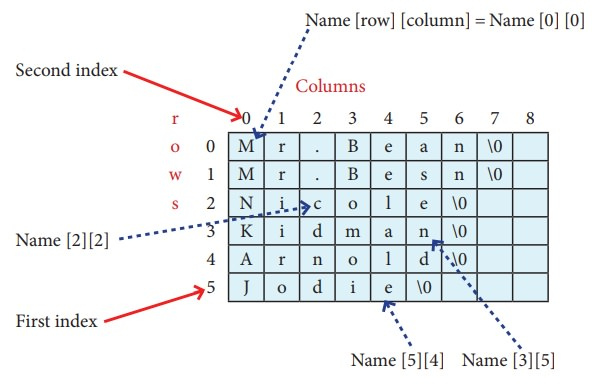Home | | Computer Science 11th std | C++: Array of strings

# C++: Array of strings

An array of strings is a two-dimensional character array.

Array of strings

An array of strings is a two-dimensional character array. The size of the first index (rows) denotes the number of strings and the size of the second index (columns) denotes the maximum length of each string. Usually, array of strings are declared in such a way to accommodate the null character at the end of each string. For example, the 2-D array has the declaration:

char Name;

In the above declaration, the 2-D array has two indices which refer to the row size and column size, that is 6 refers to the number of rows and 10 refers to the number of columns.## Initialization

For example

char Name = {"Mr. Bean", "Mr.Bush", "Nicole", "Kidman", "Arnold", "Jodie"};

In the above example, the 2-D array is initialized with 6 strings, where each string is a maximum of 9 characters long, since the last character is null.

The memory arrangement of a 2-D array is shown below and all the strings are stored in continuous locations.## C++ program to demonstrate array of strings using 2d character array

#include<iostream>

using namespace std;

int main( )

{

// initialize 2d array

char colour ={"Blue","Red","Orange", "yellow"};

// printing strings stored in 2d array

for (int i=0; i <4; i++)

cout << colour [i] << "\n";

}

### Output:

Blue

Red

Orange

Yellow

Tags : Syntax, Example Program , 11th Computer Science : Chapter 12 : Arrays and Structures
Study Material, Lecturing Notes, Assignment, Reference, Wiki description explanation, brief detail
11th Computer Science : Chapter 12 : Arrays and Structures : C++: Array of strings | Syntax, Example Program